Depending on the night, sometimes we see the Moon as a crescent, sometimes we only see half of the Moon lit, sometimes we see the Moon as a full circle. What causes this? And how do we know what the Moon will look like on any given night?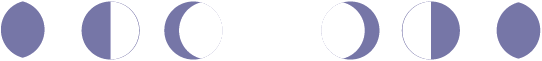The portion of the Moon that is illuminated is illuminated by the Sun. So, let’s start by taking a look at the Moon, Earth, Sun and their relationship to one another!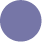new moon:

side that faces Earth is completely unlit by the Sun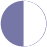quarter moon:

side that faces Earth is half lit by the Sun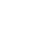full moon:

side that faces Earth is completely lit by the Sun

Here we have the Sun, the Earth, and the Moon, which orbits around the Earth.

As the Moon orbits around the Earth, the side facing the Sun is the only side that is illuminated.

The Earth can see vartying amounts of that illumination, depending on where the Moon is in its orbiting cycle.

As the Moon orbits the Earth, the side facing the Sun is illuminated. In the illustration above, the right side of the Moon is facing the Sun, so the right side of the Moon in every position of its orbit is illuminated. The side visible from the Earth contains varying amounts of that illumination, depending on where the Moon is in its orbiting cycle. This is what causes the different phases of the Moon—changes in the relative positions of the Moon, Earth, and Sun.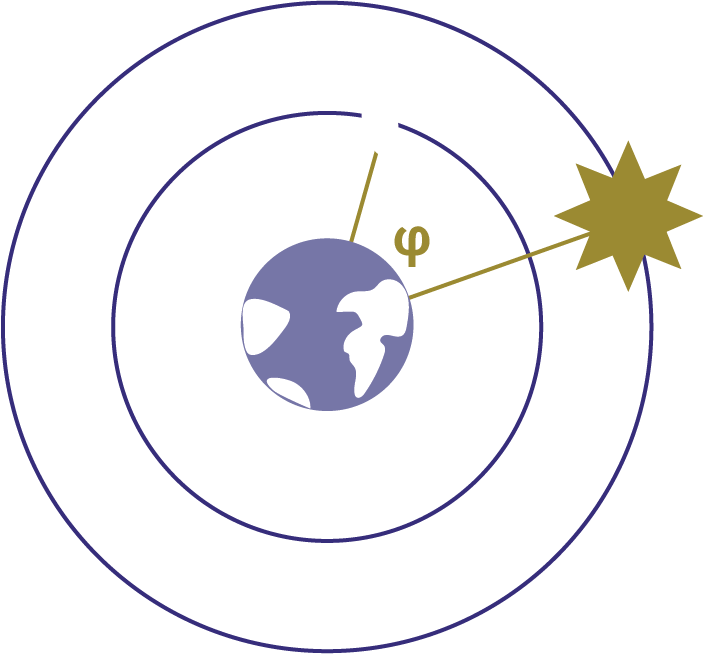Now that we've talked about the relationship between the Moon, Sun, and Earth that causes Moon phases, let’s define some angles that will be important when calculating the Moon phase. First, we’ll use the angle between the Moon and the Sun, from the Earth’s point of view, and we’ll call that phi or 𝞅.We’ll also use the angle between the Earth and the Sun, from the Moon’s point of view, and we’ll call that theta or 𝞱.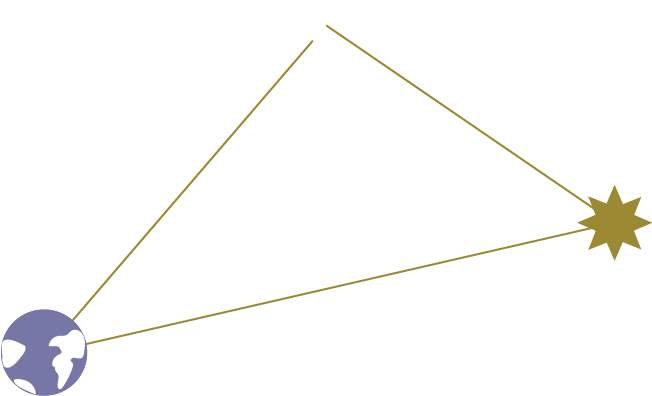a
b
𝞅
c = ?
a
known distance (Earth to Moon)
b
known distance (Earth to Sun)
𝞅
observable from Earth (angle between Sun and Moon from the POV of Earth
c
can be calculated by the Law of Cosines

The Moon, Earth, and Sun are 3 points in space, so they form a triangle.

We can fill in the lengths and angles that we know, and use trig to find the rest of the measurements.

a

b

phi

c

c

At this point it’s worth remembering that the Earth, Moon, and Sun are three points in space, so they form a triangle. Let's fill in all the information about the triangle that we know.

a One of the sides of the triangle has the length equal to the distance between the Moon and the Earth. This is a known distance, and this distance doesn’t change as the Moon revolves around the Earth and the Earth revolves around the Sun.

b The distance between the Earth and the Sun is also a known fixed distance, so we know the length of that side of the triangle.

The last side of this triangle will change in length depending on the angles between the Earth, Moon, and Sun. Do we know any of those angles?

𝞅 Earlier, we discussed phi or 𝞅, the angle that the Moon and Sun form from the point of view of the Earth. This angle is something that we can observe, given that we are on Earth. Since it's something that we can observe and measure, even though it's not a constant value, let’s count that as a known.

With this known information, we can calculate the last side, c, with the Law of Cosines.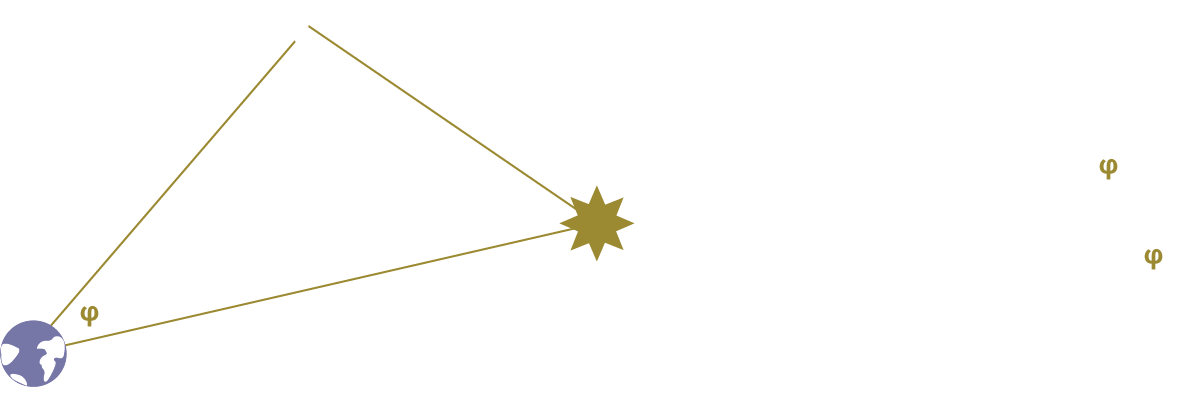Refresher on the Law of Cosines: It lets you calculate the length of an unknown side of a triangle given its opposite angle and two other sides.

So given that we know the values for 𝞅, a, and b, c, the distance between the Moon and the Sun, is the square root of a squared plus b squared minus 2ab cosine 𝞅.

Now that we know the length of c, we can calculate 𝞱, the angle between the Earth and the Sun from the Moon’s point of view. We can calculate it using the Law of Sines.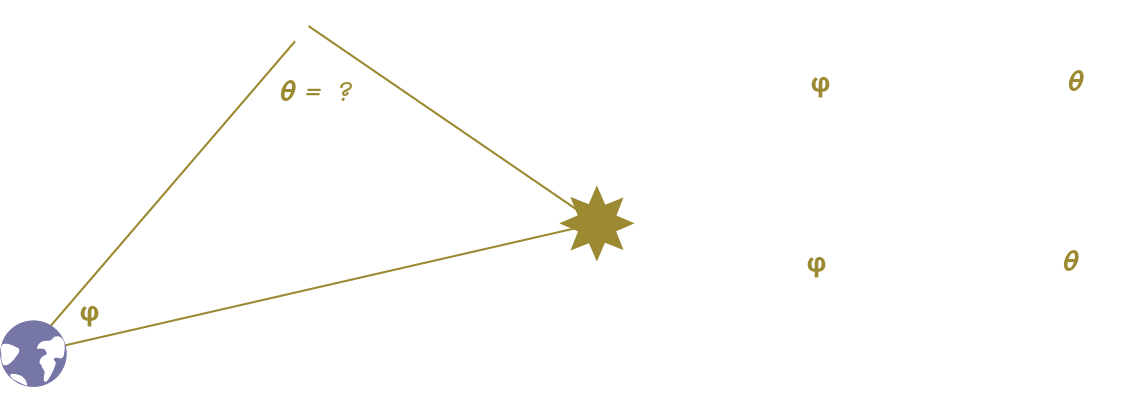Refresher on the Law of Sines: If we know all the sides of a triangle and one angle, we can calculate the rest of the angles via the Law of Sines. The Law of Sines states that the sine of an angle divided by the length of the side opposite that angle is equal to the sine of another angle divided by the length of the side opposite *that* angle.

We can substitute the value of c we calculated from the Law of Cosines and then this answer for the sine of 𝞱. Knowing the sine of 𝞱, 𝞱 is then the arcsine of that value.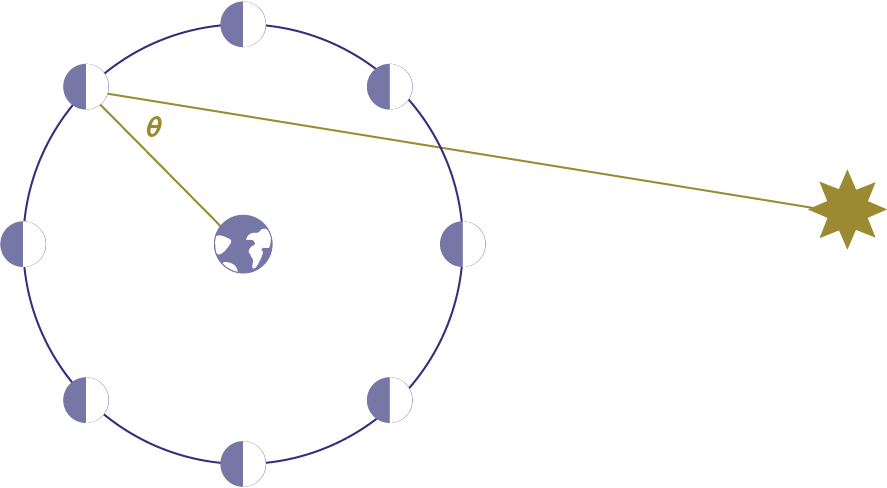area of Moon illluminated by the Sun, visible from Earth
area of Moon NOT illluminated by the Sun
side of Moon that faces away from Earth

Now we know how to calculate 𝞱, the angle between the Earth and Sun from the point of view of the Moon.

You might notice that as the Moon revolves around the Earth and changes phase, 𝞱 changes too.

Can we find an equation to describe the relationship between 𝞱 and the fraction of the Moon that’s illuminated?

To make it clearer what part of the Moon is illuminated from Earth, we’re adding a color key.

Now we know how to calculate 𝞱, the angle between the Earth and Sun from the point of view of the Moon.

You might notice that as the Moon revolves around the Earth and changes phase, 𝞱 changes too. Can we find the equation that describes the relationship between 𝞱 and the fraction of the Moon that’s illuminated?

To make it clearer what part of the Moon is illuminated from the Earth, we’re adding a color key. The white area is the part illuminated by the Sun, the grey purple area is the area not illuminated by the Sun. Then finally, the dark purple covers the side of the Moon that faces away from the Earth. So the white area that’s left is what we can see of the Moon from the Earth’s point of view.

Let’s zoom in and try to figure out how the angle of the lit area of the Moon is related to 𝞱 for an arbitrary value of 𝞱.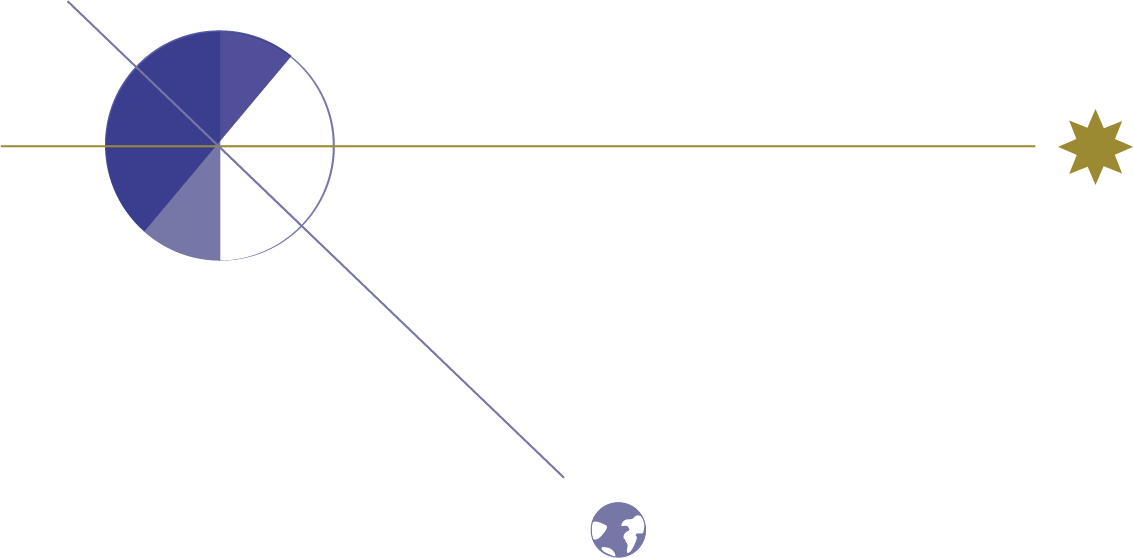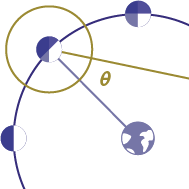𝞱
90-𝞱
90-𝞱

Let's zoom in on the previous illustration, remembering that 𝞱 is the angle between the Sun and Earth from the POV of the Moon.

Let's use this diagram to figure out how the lit area of the Moon is related to 𝞱, for an abitrary value of 𝞱.

We know the Sun lights exactly half of the Moon and forms a perpendicular with the side of the Moon that is lit, so let's mark that.

That perpendicular means that we can mark this lower lit area as having angle 90-𝞱.

The Earth also forms a perpendicular with the side of the Moon that is visible from the Earth, so we can mark that as well.

That means we can mark this upper lit area as having angle 90-𝞱, too.

So, no matter what position the Moon is in its orbit, the lit portion of the Moon has an angle of (90-𝞱) + 90 + (90-𝞱) = 180-𝞱 or 𝝿 - 𝞱 in radians.

We do know that the Sun lights exactly half of the Moon and forms a perpendicular with the side of the Moon that is lit, so we can mark that perpendicular. That perpendicular means that we can mark the bottom-most pie slice of the lit area as having angle 90-𝞱.

The Earth also forms a perpendicular with the side of the Moon that is visible from Earth, so we can mark that as well. That perpendicular means that we can mark this upper lit area as having angle 90-𝞱 as well.

So added up, the lit area of the Moon has the angle 180-𝞱 or in radians, 𝝿 - 𝞱.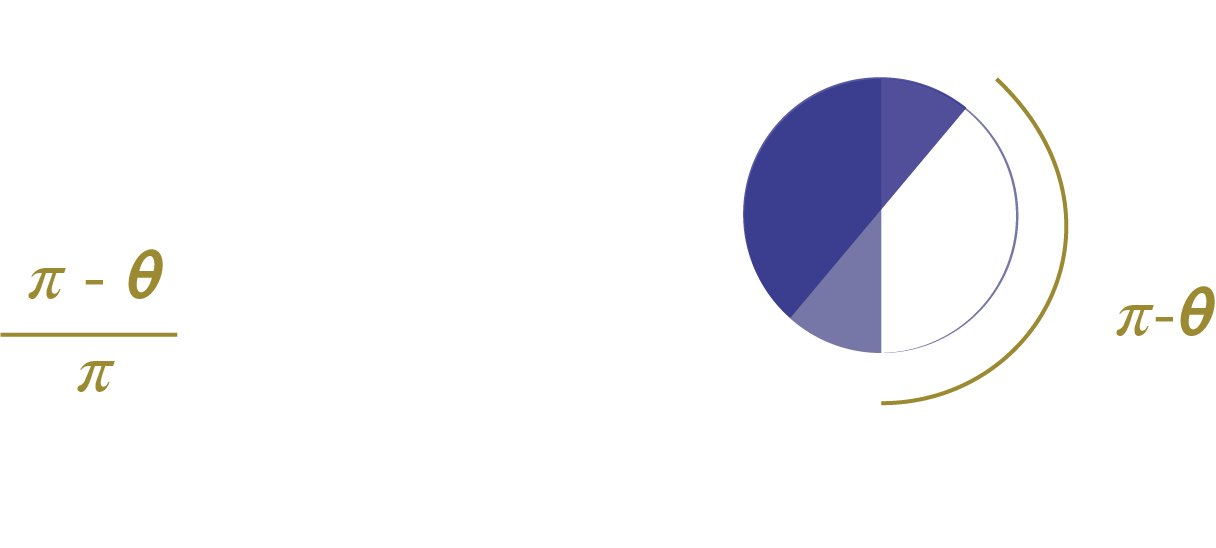From our last step, we now have the angle of the illuminated portion of the Moon, in terms of 𝞱.

So, let's use that to calculate the percentage of illumination of the Moon in terms of 𝞱.

Naively, we can calculate the fraction of the Moon illuminated as the angle illuminated, which is 𝝿 - 𝞱, divided by the total angle of the Moon visible, which is 180 degrees or 𝝿.

But, this doesn’t accurately describe the illuminated percent that we see, because the Moon is a sphere, not a circle.

We need to take into account the Moon’s curvature and how our eyes perceive that curvature.

We can naively calculate the fraction of the Moon illuminated as the angle illuminated, which is 𝝿 - 𝞱, divided by the total angle of the Moon visible, which is 180 degrees or 𝝿.

But, this doesn’t accurately describe the illuminated percent that we see, because the Moon is a sphere, not a circle. And we need to take into account the Moon’s curvature and how our eyes perceive that curvature.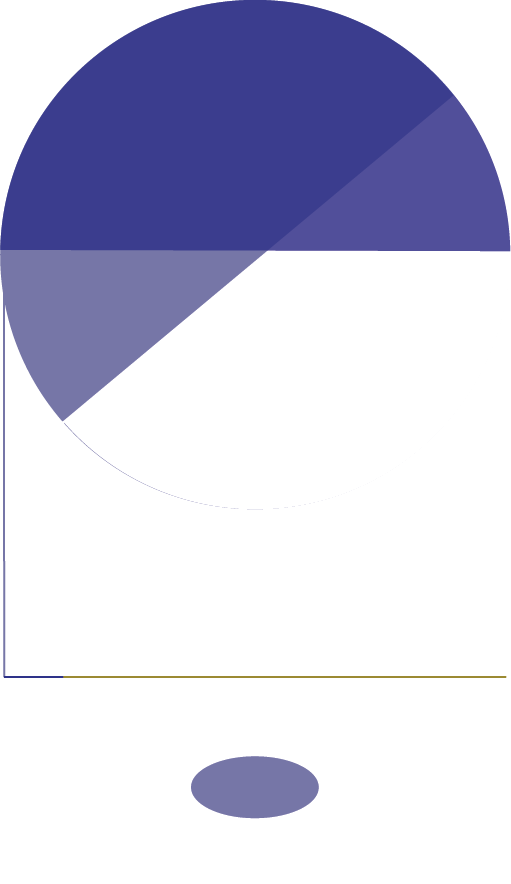𝝿-𝞱
r
r = radius of the Moonr
??
r
𝞱
r cos 𝞱
r cos 𝞱
+

Instead of using the illuminated angle or arc of the Moon, we want to use the length of the projection of the curved surface of the Moon that our eye sees.

How do we calculate the length of this projection?

We know from a previous step that the angle of the projected arc is 𝝿 - 𝞱.

Let’s also assume we know the radius of the Moon, which is a known value.

When we project r down, we see that it's a portion of the distance we care about.

What is the other portion?

The other portion of the distance is the top leg of this right triangle

With hypotenuse equal to r, the radius of the Moon

The triangle's inner angle must be 𝞱, since it forms a straight line with the angle of the lit area of the Moon, 𝝿 - 𝞱

We know from trig that length of the adjacent side to an angle in any right triangle is the hypotenuse times the cosine of that angle.

We can project down to the distance we care about.

Added together, now we know the length of the projection of the illuminated portion of the Moon.

Instead, we want to calculate the length of the projection of the curved surface of the Moon that our eye sees. How do we calculate that projection?

Let’s assume we know the radius of the Moon, which we do. The radius, r is going to be a portion of the distance we care about. What is the other portion? The other portion of the distance is a leg of this right triangle with hypotenuse r and inner angle 𝞱.

We know from the definition of the cosine that length of the adjacent side to an angle in any right triangle is the hypotenuse times the cosine of that angle. So now we know the length of that side of the right triangle, which we can project down to the distance we care about. Now we know the length of the projection of the illuminated area of the Moon as seen on Earth.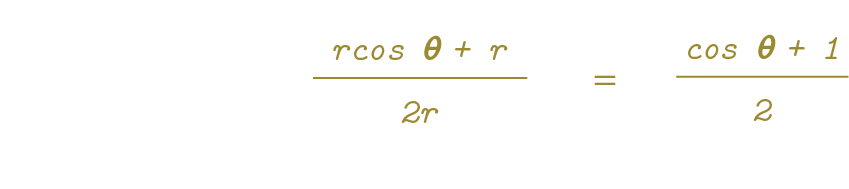To calculate the fraction illuminated we divide by the total projection, which is the diameter of the Moon. When we simplify, the Moon’s radius cancels itself out, and we’re left with cosine 𝞱 + 1 / 2. To summarize: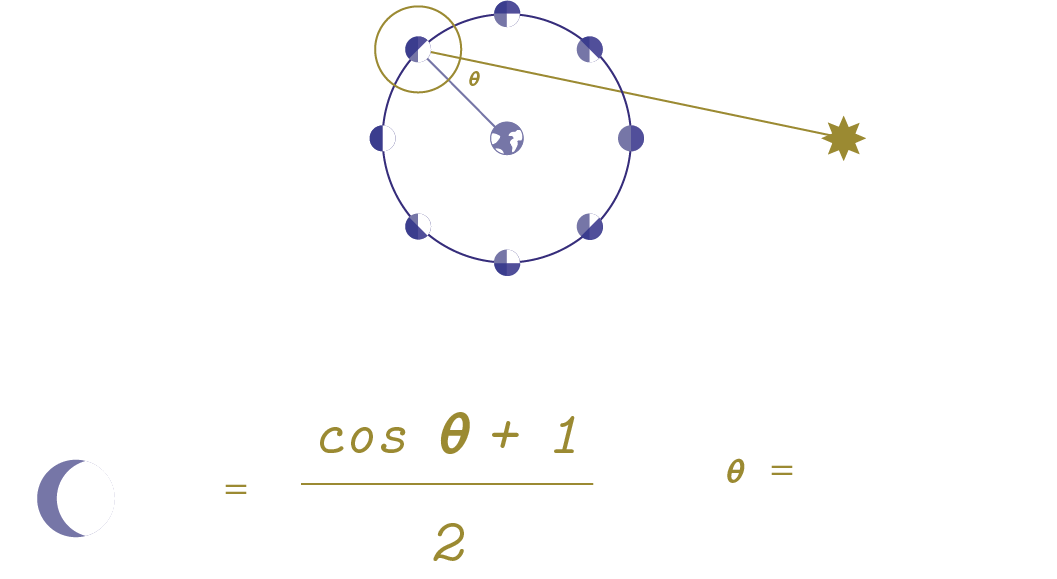Coded and illustrated with love by sailorhg. If you enjoyed this, I write and illustrate zines to explain other math and computer science concepts at bubblesort.io.